Courses

# Power Systems 3 MCQ

## 20 Questions MCQ Test Mock Test Series for SSC JE Electrical Engineering | Power Systems 3 MCQ

Description
This mock test of Power Systems 3 MCQ for Electrical Engineering (EE) helps you for every Electrical Engineering (EE) entrance exam. This contains 20 Multiple Choice Questions for Electrical Engineering (EE) Power Systems 3 MCQ (mcq) to study with solutions a complete question bank. The solved questions answers in this Power Systems 3 MCQ quiz give you a good mix of easy questions and tough questions. Electrical Engineering (EE) students definitely take this Power Systems 3 MCQ exercise for a better result in the exam. You can find other Power Systems 3 MCQ extra questions, long questions & short questions for Electrical Engineering (EE) on EduRev as well by searching above.
QUESTION: 1

### Which of the following is not the correct statement regarding direct current transmission?

Solution:

1) Corona effect is less in DC transmission compared to AC transmission

2) Voltage regulation is better in case of DC transmission

3) Skin effect is absent in DC transmission, it is more predominant in AC transmission

4) Continuous power losses does not happen in DC transmission

QUESTION: 2

### An ac current passing through a conductor distributes _________ throughout the cross section and is frequency_________

Solution:

Skin effect is the tendency of a high-frequency alternating current to flow through only the outer layer of a conductor. It is more predominant in AC transmission.

When an AC current is passing through a conductor distributes non-uniformly throughout the conductor. And it is dependent of frequency, it is more in high frequency transmission.

QUESTION: 3

### The characteristic impedance of a loss less line in called:

Solution:

The characteristic impedance of a lossless line is called surge impedance. It is given by

Surge impedance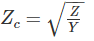Where Z is impedance and Y is admittance

QUESTION: 4

For flat voltage profile system, voltage regulation is:

Solution:

For flat voltage system, receiving end voltage is equal to sending end voltage.

Vs = VR

Voltage regulation =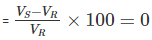QUESTION: 5

Corona discharge is generally ________ in colour:

Solution:

The phenomenon of electrical discharge occurring in transmission line for high values of voltage is known as the corona effect. If the voltage across the lines still gets increased the glow becomes more and more intense along with hissing noise, inducing high power loss into the system. This is generally in violet or blue color.

QUESTION: 6

The non-uniform distribution of voltage across the units in a string of suspension insulators is due to:

Solution:

Important points regarding the voltage distribution over a string of suspension insulators:

1) Due to the presence of shunt capacitor, the voltage across the suspension insulators does not distribute itself uniformly across the each disc.

2) The voltage across the nearest disc to the conductor is maximum than others disc.

3) The unit nearest to the conductor is under maximum electrical stress and is likely to be punctured.

4) In the case of D.C voltage, the voltage across each unit would be the same. It is because insulator capacitance are ineffective for D.C.

QUESTION: 7

What does 100% string efficiency imply?

Solution:

The Ratio of the voltage across the whole string to the product of numbers of discs and the voltage across the disc nearest to the conductor is known as string efficiency.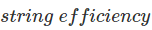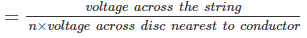The greater the string efficiency, the more uniform is the voltage distribution in the each disc insulator. 100% string efficiency implies that the potential across each disc is same.

QUESTION: 8

The sag of a transmission line depends upon:

Solution:

Sag is defined as the difference in level between points of supports and the lowest point on the conductor.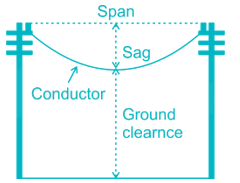Sag of conductor’s between two poles can be determined by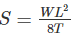Where, S is the sag of conductors

W is the weight per unit length of the conductor

L is the length of span

T is the tension in the conductor

QUESTION: 9

Armouring in cable is used to protect the cable from:

Solution:

Armouring provides earthing shield to the current carrying conductors as well as it is also used for earthing purpose of the cable for safety. When there is any insulation failure in the conductor, the fault current gets enough paths to flow through the armour if it is properly earthed. And it also provides extra mechanical protection and strength to cable.

QUESTION: 10

Solution:

In radial distribution system, different feeders radially came out from the substation and connected to the primary of distribution transformer.

It is cheapest to build, and is widely used in sparsely populated areas. A radial system has only one power source for a group of customers. A power failure, short-circuit, or a downed power line would interrupt power in the entire line which must be fixed before power can be restored.

Hence it is used when energy is to be produced on low potential.

QUESTION: 11

Which of the following is most commonly used is secondary distribution?

Solution:

1-phase 2-wire: In this, one of the two wires is earthed or mid-point of phase winding is earthed. This system is used for very short distances

1-phase 3-wire: This system is identical in principle with 3-wire dc distribution system. The neutral wire is centre-tapped from the secondary winding of the transformer and earthed. This system is also called as split-phase electricity distribution system

2-phase 3-wire: In this system, the neutral wire is taken from the junction of two phase windings whose voltages are in quadrature with each other. The voltage between neutral wire and either of the outer phase wires is V whereas, the voltage between outer phase wires is √2V. As compared to a two-phase 4-wire system, this system suffers from voltage imbalance due to unsymmetrical voltage in the neutral

3-phase 4-wire: This system uses star connected phase windings and the fourth wire or neutral wire is taken from the star point. If the voltage of each winding is V, then the line-to-line voltage (line voltage) is √3V and the line-to-neutral voltage (phase voltage) is V. This type of distribution system is widely used in India for secondary distribution

QUESTION: 12

For a fault at terminals of the synchronous generator fault current to be maximum:

Solution:

For a line to ground fault,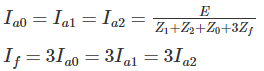The fault current is three times the line current.

QUESTION: 13

Fusing current is the :

Solution:

A fuse is an electrical safety device that operates to provide overcurrent protection of an electrical circuit. Its essential component is a metal wire or strip that melts when too much current flows through it, thereby interrupting the current. The minimum current at which the fuse element will melt is called as fusing current

QUESTION: 14

________ does not have any repairable component:

Solution:

MCB (miniature circuit breaker) is an electromechanical device which guards an electrical circuit from an over current that may effect from short circuit, overload or imperfect design. It doesn’t have any repairable component.

MCB is substituting the rewirable switch-fuse units for low power domestic and industrial applications in a very quick manner. In wiring system, the MCB is a blend of all three functions such as protection of short circuit, overload and switching. Protection of overload by using a bimetallic strip and short circuit protection by using solenoid

QUESTION: 15

Arcing contacts in a circuit breaker is usually made of:

Solution:

In a circuit breaker, the main contacts are usually made up of copper and conduct current in closed positions. Circuit breakers have low contact resistance and they are silver plated. The arcing contacts are solid, resistant to heat and are made up of copper tungsten alloy.

QUESTION: 16

In a circuit breaker, ionisation is not facilitated by:

Solution:

In a circuit breaker, ionisation is facilitated by

1) Increase of mean free length

2) Increase of filed strength

3) High temperature of surrounding medium

QUESTION: 17

Which of the following relay, operates when current exceeds a present value?

Solution:

Over current relay: It operates or picks up when its current exceeds a predetermined value (setting value). It protects electrical power systems against excessive currents which are caused by short circuits, ground faults, etc.

Differential relay: It is defined as the relay that operates when the phase difference of two or more identical electrical quantities exceeds a predetermined amount. The differential relay works on the principle of comparison between the phase angle and magnitude of two or more similar electrical quantities.

Static relay: The relay which does not contain any moving parts is known as the static relay. In such type of relays, the output is obtained by the static components like magnetic and electronic circuit etc.

QUESTION: 18

A pitot relay is used for:

Solution:

Pitot relay is to be attached to a pipe that connects a transformer body and a conservator in order to detect any trouble and to protect the transformer from any accident. It has slight failure contacts to detect gas accumulation as well as serious failure contacts to detect rapid flow of insulation liquid. It is sued for high speed tripping.

QUESTION: 19

If some reserve is available in a power plant,

Solution:

Plant capacity factor: It is the ratio of the average demand on the station and the maximum installed capacity of the station

Plant use factor: It is the ratio of kW generated to the product of the plant capacity and the number of hours for which the plant was in operation

Reserve capacity is the difference between plant capacity and maximum demand

Reserve capacity = plant capacity - maximum demand

Given that some reserve capacity is available, that means plant capacity is greater than maximum demand.

Capacity factor = load factor × use factor

Load factor is always less than one. Hence plant’s use factor is always greater than its capacity factor

QUESTION: 20

Two areas A and B have equal connected loads however load diversity in area A is more than in B then:

Solution:

Diversity factor is the ratio of the sum of the maximum demands of a group of consumers and the simultaneous maximum demand of the group of consumers

Diversity factor = connected load/maximum demand

Given that connected load is same for both A and B

Then, diversity factor is inversely proportional to maximum demand

Given that diversity factor in area A is more hence maximum demand of A is less than maximum demand of B